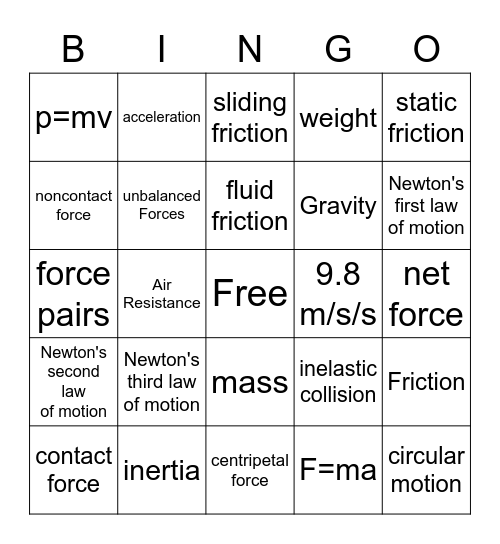# Forces and MotionThis bingo card has a free space and 29 words: momentum, inertia, sliding friction, law of conservation of momentum, static friction, p=mv, unbalanced Forces, fluid friction, 9.8 m/s/s, Friction, contact force, Air Resistance, F=ma, circular motion, force pairs, Balanced Forces, centripetal force, inelastic collision, Newton's first law of motion, Newton's second law of motion, Newton's third law of motion, Force, Gravity, net force, noncontact force, acceleration, mass, weight and elastic collision.

## Play Online